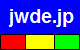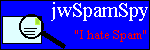joewein.de LLC
fighting spam and scams on the Internet
 Try our spam filter! Free trial for 30 daysHome About Us Spam 419/Nigeria Fraud Contact

"419" Scam – Advance Fee / Fake Lottery Scam

The so-called "419" scam is a type of fraud dominated by criminals from Nigeria and other countries in Africa. Victims of the scam are promised a large amount of money, such as a lottery prize, inheritance, money sitting in some bank account, etc.

Victims never receive this non-existent fortune but are tricked into sending their money to the criminals, who remain anonymous. They hide their real identity and location by using fake names and fake postal addresses as well as communicating via anonymous free email accounts and mobile phones.

Keep in mind that scammers DO NOT use their real names when defrauding people.
The criminals either abuse names of real people or companies or invent names or addresses.
Any real people or companies mentioned below have NO CONNECTION to the scammers!

Read more about such scams here or in our 419 FAQ. Use the Scam-O-Matic to verify suspect emails.

• This email uses a separate reply address that is different from the sender address. Spammers use this to get replies even when the original spam sending accounts have been shut down. Also, sometimes the sender addresses are legitimate looking but fake and only the reply address is actually an email account controlled by the scammers.
• The following phrases in this message should put you on alert:
• "00,000.00" (they want you to be blinded by the prospect of quick money, but the only money that ever changes hands in 419 scams is from you to the criminals)
• This email message is a 419 scam. Please see our 419 FAQ for more details on such scams.
• This email lists free webmail addresses. Use of such addresses is typical for scams. Lotteries, banks and any but the smallest of companies do not normally use such addresses. Criminals use them to anonymously send and receive email at Internet cafes.
• manuelfranco188@yahoo.com (Yahoo; can be used from anywhere worldwide)
• vict= orious. contact me urgently for claims via: manuelfranco188@yahoo.com. watc= h my full interview here: (Yahoo; can be used from anywhere worldwide)

Fraud email example:

 From: "Manuel Franco" Reply-To: manuel_franco@rediffmail.com Date: Thu, 02 Sep 2021 05:13:23 -0700 Subject: [SPAM] you have a donation of \$2,800,000.00. Hello, I am Manuel Franco, you have a donation of \$2,800,000.00. I won the = \$768 million Powerball lottery on March 27, 2019, I am donating part of it = to five lucky people and Ten Charity organizations. You email came out vict= orious. Contact me urgently for claims via: manuelfranco188@yahoo.com. watc= h my full interview here: https://youtu.be/R_pDrt1np1U. My reasons for this= donation is because i know how it feels to have nothing. I am doing my bes= t to put a smile in the faces of everyone, in any way i can. = =60A8=597D=FF0C=6211=662F Manuel Franco=FF0C=60A8=6536=5230=4E86 2,800,000= .00 =7F8E=5143=7684=6350=6B3E=3002 =6211=5728 2019 =5E74 3 =6708 27 =65E5= =8D62=5F97=4E86 7.68 =4EBF=7F8E=5143=7684=5F3A=529B=7403=5F69=7968=FF0C= =6211=5C06=5176=4E2D=7684=4E00=90E8=5206=6350=8D60=7ED9=4E94=4E2A=5E78=8FD0= =7684=4EBA=548C=5341=4E2A=6148=5584=7EC4=7EC7=3002 =60A8=7684=7535=5B50= =90AE=4EF6=53D6=5F97=4E86=80DC=5229=3002 =901A=8FC7=4EE5=4E0B=65B9=5F0F= =7D27=6025=8054=7CFB=6211=8FDB=884C=7D22=8D54=FF1Amanuelfranco188@yahoo.com= =3002 =5728=8FD9=91CC=89C2=770B=6211=7684=5B8C=6574=91C7=8BBF=FF1Ahttps://y= outu.be/R_pDrt1np1U=3002 =8FD9=6B21=6350=8D60=7684=539F=56E0=662F=56E0=4E3A= =6211=77E5=9053=4EC0=4E48=90FD=6CA1=6709=7684=611F=89C9=3002 =6211=6B63= =5728=5C3D=6211=6240=80FD=FF0C=7528=6211=6240=62E5=6709=7684=4E00=70B9=70B9= =8BA9=8FD9=4E2A=4E16=754C=53D8=5F97=66F4=7F8E=597D=3002

Anti-fraud resources: